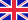•# Pendulum (mathematics)

Autor: Lambert M. Surhone

The mathematics of pendulums are in general quite complicated. Simplifying assumptions can be made, which in the case of a simple pendulum allows the equations of motion to be solved analytically for small-angle oscillations. Simple gravity pendulum Trigonometry... Viac o knihe

Na objednávku, dodanie 2-4 týždne

29.75 €

bežná cena: 35.00 €

## O knihe

The mathematics of pendulums are in general quite complicated. Simplifying assumptions can be made, which in the case of a simple pendulum allows the equations of motion to be solved analytically for small-angle oscillations. Simple gravity pendulum Trigonometry of a simple gravity pendulum. A simple pendulum is an idealisation, working on the assumption that: . The rod or cord on which the bob swings is massless, inextensible and always remains taut; . Motion occurs in a 2-dimensional plane, i.e. the bob does not trace an ellipse. . The motion does not lose energy to friction.

• Vydavateľstvo: Betascript Publishers
• Rok vydania: 2009
• Formát: Paperback
• Rozmer: 220 x 150 mm
• Jazyk: Anglický jazyk
• ISBN: 9786130305208

Generuje redakčný systém BUXUS CMS spoločnosti ui42.